Next: Feasibility of measurements Up: Results Previous: Measurement of vertical displacements

### Measurement of transverse rotation (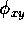)

Since the beams are very flat because R=85.5 for JLC-1, a small transverse rotation () of the beam causes a significant loss of luminosity. This effect is enhanced by the presence of the crossing angle. Actually, the luminosities were estimated using ABEL to be 0.93-, 0.80- and 0.55-times the nominal one for=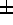0.005, 0.01 and 0.02 radian, respectively, where + and - are for beam-1 and beam-2, respectively. Even for rotation in the same direction, i.e.  no relative rotation between two beams, similar luminosity losses were obtained. On the other hand, in a head-on collision the luminosity loss is only 20% at most, even for=0.02.

First for the opposite rotation the right half of beam-1 with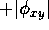traverses above beam-2 with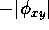during a collision, while the left half traverses below beam-2. Therefore, the azimuthal distribution from the right (left) half of beam-1 has more (less) particles at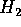than at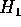. If two beams collide head-on, no such up-down asymmetry is expected in the total distribution, since the right- and left-hand sides cancel each other. However, under the presence of a horizontal crossing angle (see Fig. 2), particles on the right-hand side are deflected more than those on the left. We therefore expect an up-down asymmetry of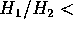1. The results of the simulation agree with our expectation, as shown in Fig.10. The ratios of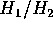were obtained to be 0.99, 0.92 and 0.78 for=0.005,0.01 and0.02, respectively.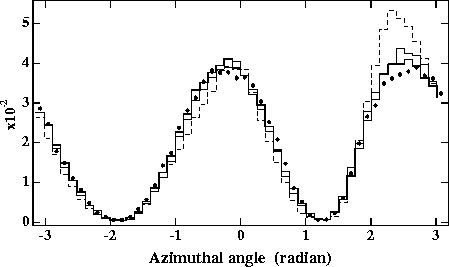Figure 10:   Azimuthal angular distributions measured for 6< r < 7cm at z=+1m for various transverse rotations compared with the nominal one(solid circles). The signs of the rotations in xy plane are opposite for two beams, i.e.=0.005(solid line),0.01(dotted one),0.02(dashed one) radian. The distributions are normalized by the total number of particles.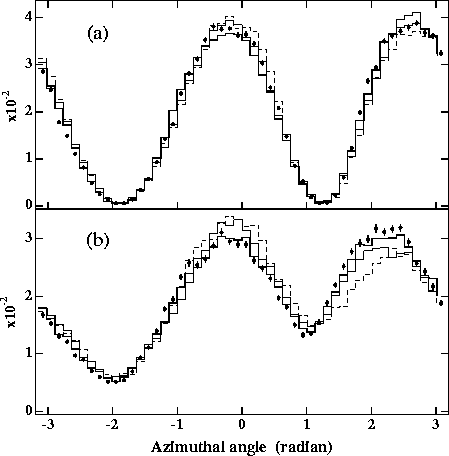Figure 11:   Azimuthal angular distributions measured for (a) 6< r < 7cm and (b) 5< r < 6cm at z=+1m for various rotations compared with the nominal one(solid circles). The magnitudes of the rotations are=+ 0.005(solid line), +0.01(dotted one), +0.02(dashed one) for both beams. The distributions are normalized by the total number of particles.

Secondly, for the same rotation case, beam-1 traverses beam-2 from its top(bottom) surface to its bottom(top) surface if the sign of the rotation is positive(negative). We may expect an enhancement at the down(up) side due to the crossing angle from the same arguments as in the case of the opposite rotation. The simulation shows slight enhancements in the distribution for 6< r < 7cm, as can be seen in Fig.11(a), while the distribution for smaller radii, 5< r < 6cm, has clear up-down asymmetries for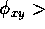0.01, as shown in Fig.11(b). The reason for this radial dependence can be explained based simply upon geometrical considerations as follows. During a collision the vertical displacement between the two beams is approximately a linear function of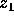and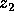(Fig.2) as well as. The particles measured for 6< r < 7cm are really created in the central region of the collision,i.e.  within about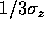in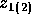, where the vertical distance is very close to zero. The particles measured at smaller radii should be created over a wider region alongwith a significant amount of vertical displacement. Thus, the sensitivity againstin up-down asymmetries increases as r becomes smaller. However, there is a certain limitation because of the poor monochromaticity of particles energies at smaller r, as can be seen in Fig.5(a).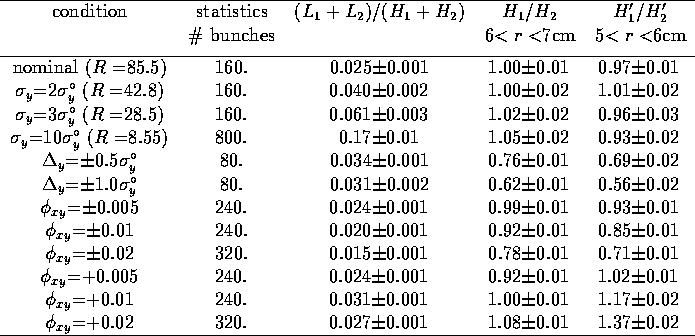Table 1: Summary of the results obtained by ABEL for JLC-I at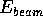=250GeV. The errors are only statistical, corresponding to the number of bunch crossings listed in the second column. The conditions in the first column show only changed beam parameters, assuming that the others are the same as the nominal one. These conditions are for both beams, unless specified. The upper and lower signs in the first column correspond to beam-1 and beam-2, respectively. In the last column,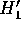= -0.3770.251 and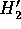= 2.0102.638 according to a movement of the second peak in the azimuthal angular distributions for 5< r <6cm.

Finally, we summarize all of the results in Tab.1. Here, although we show the cases of particular signs of displacements and rotations, the results for the opposite signs are simply obtained by exchangingand. And although both the vertical displacements and the transverse rotations produce up-down asymmetries in, the separation between them is evident, as can be seen in Figs.9,10 and 11.Next: Feasibility of measurements Up: Results Previous: Measurement of vertical displacements

Toshiaki Tauchi
Sat Dec 21 00:34:16 JST 1996